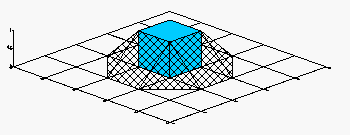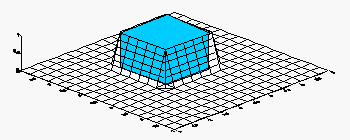# Calculating the Volume of a Grid with NoData Values

Surfer can be used to calculate the volume between two grid surfaces or between a grid surface and a horizontal plane. The accuracy of the volume calculation increases as the number of grid nodes in the grid file increases. When one of the grid surfaces contains NoData values, or the area of interest is a subset of the entire grid, additional error is introduced. This error can be minimized by specifying more grid nodes during the gridding operation.

Consider the way Surfer calculates the volume of a cube that is 1 foot x 1 foot x 1 foot. Surfer cannot generate a surface that is a perfect cube since each XY coordinate can only have a single Z value. A diagonal line is drawn from the top surface to the lower with a width of one grid unit. Using a grid with a one-foot grid line spacing, the resulting surface looks like a pyramid with the top chopped off.A cube with a coarse grid. Volume error is increased.A cube with a finer grid. Volume error is reduced.